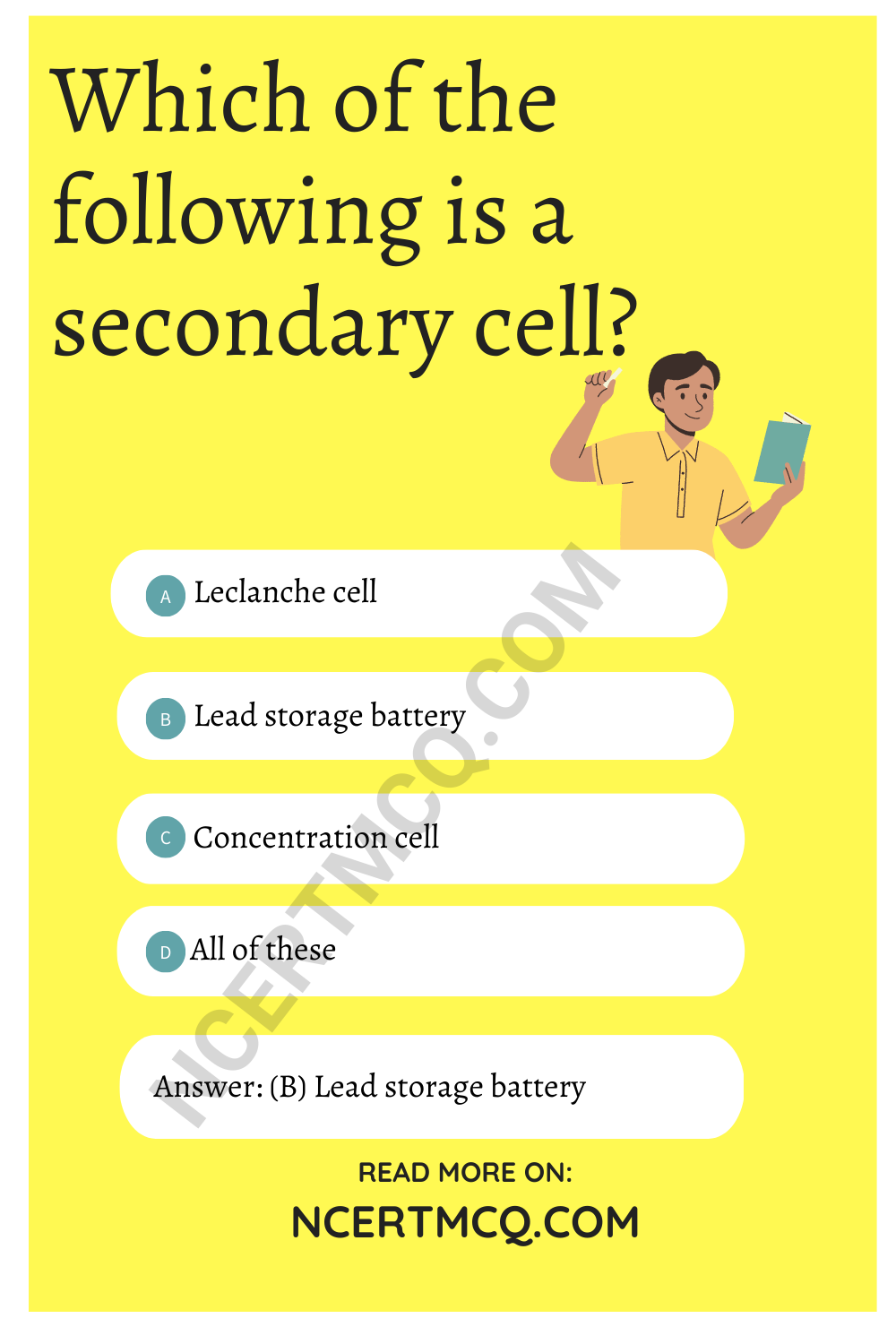Check the below NCERT MCQ Questions for Class 12 Chemistry Chapter 3 Electrochemistry with Answers Pdf free download. MCQ Questions for Class 12 Chemistry with Answers were prepared based on the latest exam pattern. We have provided Electrochemistry Class 12 Chemistry MCQs Questions with Answers to help students understand the concept very well.

## Electrochemistry Class 12 MCQs Questions with Answers

Electrochemistry MCQ Class 12 Question 1.
If 96500 coulomb electricity is passed through CuSO4 solution, it will liberate
(a) 63.5 gm of Cu
(b) 31.76 gm of Cu
(c) 96500 gm of Cu
(d) 100 gm of Cu

Answer: (b) 31.76 gm of Cu

Electrochemistry Class 12 MCQ Question 2.
Fused NaCl on electrolysis gives ………….. on cathode.
(a) Chlroine
(b) Sodium
(c) Sodium amalgam
(d) Hydrogen

MCQ On Electrochemistry Class 12 With Answers Question 3.
The standard electrode potentials for the half cell reactions are:
Zn → Zn2-– 2e E° = 0.76 V
Fe → Fe2- + 2 E° = -0.41 V
The emf of the cell reaction
Fe2- + Zn → Zn2- + Fe is
(a) -0.35 V
(b) +0.35 V
(c) -1.17 V
(d) +1.17 V

Class 12 Chemistry Chapter 3 MCQ Question 4.
Which of the following is a secondary cell?
(a) Leclanche cell
(c) Concentration cell
(d) All of theseElectrochemistry MCQ Questions Class 12 Question 5.
For a certain redox reaction, E° is positive. This means that
(a) ΔG° is positive, K is greater than 1
(b) ΔG° is positive, K is less than 1
(c) ΔG° is negative, K is greater than 1
(d) ΔG° is negative, K is less than 1

Answer: (c) ΔG° is negative, K is greater than 1

Electrochemistry MCQ Class 12 Question 6.
Cell reaction is spontaneous, when
(a) E$$_{red}^{0}$$ is negative
(b) ΔG° is negative
(c) E$$_{oxid}^{0}$$ is Positive
(d) ΔG° is positive

MCQ Of Electrochemistry Class 12 Question 7.
Equilibrium constant K is related to E$$_{cell}^{0}$$ and not E$$_{cell}$$ because
(a) E$$_{cell}^{0}$$ is easier to measure than E$$_{cell}$$
(b) E$$_{cell}$$ becomes zero at equilibrium point but E$$_{cell}^{0}$$ remains constant under all conditions
(c) at a given temperature, E$$_{cell}$$ changes hence value of K can’t be measured
(d) any of the terms E$$_{cell}$$ or E$$_{cell}^{0}$$ can be used

Answer: (b) E$$_{cell}$$ becomes zero at equilibrium point but E$$_{cell}^{0}$$ remains constant under all conditions

MCQ Electrochemistry Class 12 Question 8.
Molar conductivity of 0.15 M solution of KCl at 298 K, if its conductivity of 0.0152 S cm-1 w ill be
(a) 124 Ω-1 cm² mol-1
(b) 204 Ω-1 cm² mol-1
(c) 101 Ω-1 cm² mol-1
(d) 300 Ω-1 cm² mol-1

Answer: (c) 101 Ω-1 cm² mol-1

MCQ Questions For Class 12 Chemistry Chapter 3 Question 9.
Electrical conductance through metals is called metallic or electronic conductance and is due to the movement of electrons. The electronic conductance depends on
(a) the nature and structure of the metal
(b) the number of valence electrons per atom
(c) change in temperature
(d) all of these

MCQ Of Chapter 3 Chemistry Class 12 Question 10.
The specific conductivity of N/10 KCl solution at 20°C is 0.0212 ohm-1 cm-1 and the resistance of the cell containing this solution at 20°C is 55 ohm. The cell constant is
(a) 3.324 cm-1
(b) 1.166 cm-1
(c) 2.372 cm-1
(d) 3.682 cm-1

MCQ On Electrochemistry Class 12 Pdf Question 11.
Faraday’s law of electrolysis is related to
(a) Atomic number of cation
(b) Speed of cation
(c) Speed of anion
(d) Equivalent weight of electrolyte

Answer: (d) Equivalent weight of electrolyte

Class 12 Electrochemistry MCQ Question 12.
The molar conductivity is maximum for the solution of concentration
(a) 0.004 M
(b) 0.002 M
(c) 0.005 M
(d) 0.001 M

MCQ Questions On Electrochemistry Question 13.
Units of the properties measured are given below. Which of the properties has been not matched correctly?
(a) Molar conductance = Sm2 mol-1
(b) Cell constant = m-1
(c) Specific conductance of = S m²
(d) Equivalence conductance = S m² (g eq)-1

Answer: (c) Specific conductance of = S m²

MCQ On Electrochemistry Class 12 Question 14.
How long would it take to deposit 50 g of Al from an electrolytic cell containing Al2O3 using a current of 105 ampere?
(a) 1.54 h
(b) 1.42 h
(c) 1.32 h
(d) 2.15 h

The charge required for reducing 1 mole of MnO$$_{4}^{-}$$ to Mn2- is
(a) 1.93 × 105 C
(b) 2.895 × 105 C
(c) 4.28 × 105 C
(d) 4.825 × 105 C

Answer: (d) 4.825 × 105 C

Question 16.
How much electricity in in terms of Faraday is required to produce 100 g of Ca from molten CaCl2?
(a) 1F
(b) 2F
(c) 3F
(d) 5F

Question 17.
If a current of 1.5 ampere flows through a metallic wire for 3 hours, then how many electrons would flow through the wire?
(a) 2.25 × 1022 electrons
(b) 1.13 × 1023 electrons
(c) 1.01 × 1023 electrons
(d) 4.5 × 1023 electrons

Answer: (c) 1.01 × 1023 electrons

Question 18.
How many coulombs of electricity is required to reduce 1 mole of Cr2O$$_{7}^{2-}$$ in acidic medium?
(a) 4 × 96500 C
(b) 6 × 96500 C
(c) 2 × 96500 C
(d) 1 × 96500 C

Answer: (b) 6 × 96500 CQuestion 19.
A current of 1.40 ampere is passed through 500 mL of 0.180 M solution of zinc sulphate for 200 seconds. What will be the molarity of Zn2+ions after deposition of zinc?
(a) 0.154 M
(b) 0.177 M
(c) 2 M
(d) 0.180 M

Question 20.
How much time is required to deposit 1 × 10-3 cm thick layer of silver (density of 1.05 g cm-3) on a surface of area 100 cm² by passing a current of 5 A through AgNO3 solution?
(a) 125 s
(b) 115 s
(c) 18.7 s
(d) 27.25 s